# Varistor/Voltage Dependent Resistor Circuit with Working

An electrical resistor can be defined as a basic component of electrical and electronics circuits. Resistors are basically used for controlling the electrical parameters (voltage and current) in a circuit using the property of resistor termed as resistance.

There are different types of resistors such as fixed resistors carbon (composition resistors, carbon film resistors, metal oxide film resistors, wire wound resistors, thin film resistors, metal film resistors),  & variable resistors (wire-wound variable resistors, potentiometers, cermet variable resistors, rheostats, conductive plastic variable resistors), leaded (all resistors with leads) & non-leaded resistors (surface mount resistors), and special type of resistors such as pencil resistor, light dependent resistor (LDR), voltage dependent resistor (VDR), and so on.

Here, in this article let us discuss in detail about varistor, varistor working, varistor circuit, varistor function, and varistor application. But, primarily we must know what is varistor.

## What is Varistor?

A special type of resistor whose resistance can be varied by varying the applied voltage is termed as a voltage dependent resistor (VDR) and is also simply called as varistor. It is a non-linear semiconductor element and its name is obtained from the words variable resistor.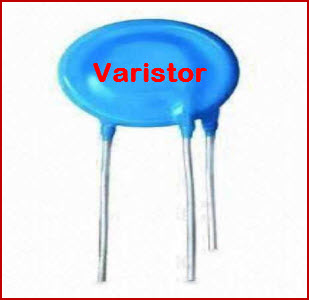These varistors are used as protective devices to avoid excess amounts of transient voltages such that to protect the components of the circuits and control the operating conditions of the circuits. The design and size of varistor is almost similar to a capacitor and hence it will be a bit confused to identify between a varistor and capacitor.

### Varistor Working

In general circuit operating conditions, the varistor exhibits high resistance. Whenever the transient voltages starts increasing then the resistance of the varistor starts decreasing. Thus, as it starts conducting and the transient voltage is clamped to a safe level.

Even though there are various types of various, metal oxide varistor is most frequently used in the practical varistor application. In most the practical applications varistor function is to protect the circuit from the excessive transient voltages. These transient voltages are generally caused due to electrostatic discharges and lightning surges.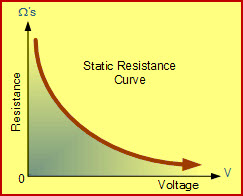Voltage vs Resistance Curves of Varistor

The varistor working can be easily understood by having a glance at varistor static resistance curve, which is drawn between resistance of VDR (voltage dependent resistor or varistor) and the applied voltage. The graph shown above indicates that during normal operating voltage (say low voltage) the resistance very high and if the voltage applied exceeds rated value of varistor, then its resistance starts decreasing.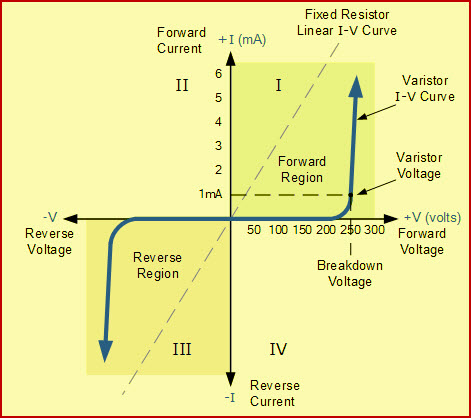V-I Characteristics of Varistor

The varistor V-I characteristics shown in the above figure represent that small change in applied voltage causes a significant amount of change in current. As shown in the V-I characteristics, it acts as two Zener diodes connected back to back and operates in both quadrants one and three (both directions).

The voltage level at which the current flowing through the varistor is 1mA, at this level varsitors start changing their state from insulating to conducting. This is because, whenever the applied voltage is greater than or equal to rated voltage, then the avalanche effect of semiconductor material of varistors turns them into conductors by reducing the resistance.

Thus, even though there is a rapid rise of small leakage current the voltage will be just above the rated value. Thus, the varistor function will regulate the transient voltage itself based on the applied voltage.

#### Varistor Application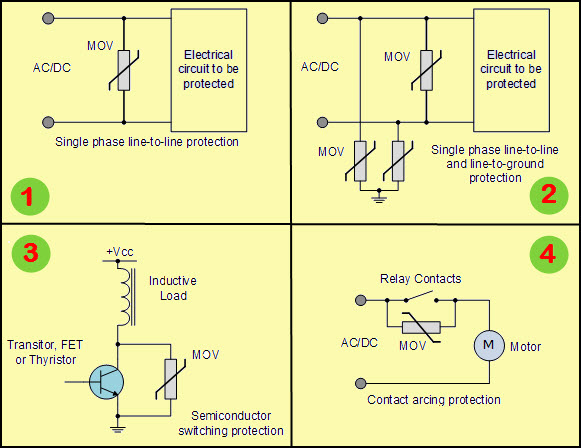Varistor Application with Varistor Circuit

The above figure shows varistor application in various power systems protection systems. Each varistor application is explained below with varistor circuit.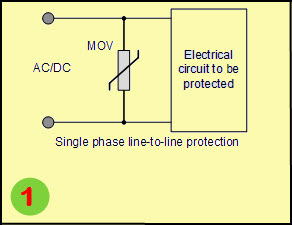Varistor Circuit for Single Phase Line to Line Protection

The varistor circuit shown in the above figure1 represents the single phase line to line protection system. In this system, the varistor is connected across the electrical circuit which is intended to be protected. If any voltage transient occurs across the line to line terminals of electrical circuit, then the voltage dependent resistor will reduces its resistance and thus protects the electrical circuit.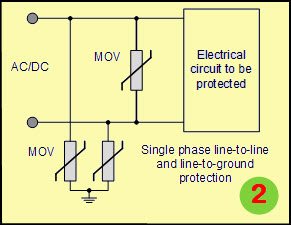Varistor Circuit for Single Phase Line to Line and Line to Ground Protection

The varistor circuit shown in the above figure2 represents the single phase line to line and line to ground protection system. In this system, the varistor is connected across the electrical circuit and to the supply terminals which are intended to be protected. Similar to the above circuit, here in this circuit voltage dependent resistors are connected across both the line to line and line to ground terminals.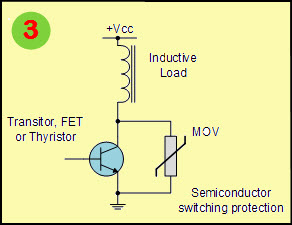Varistor Circuit for Semiconductor Switching Protection

The varistor circuit shown in the above figure3 represents the semiconductor switching protection system. In this system, the varistor is connected across the semiconductor switching device (such as transistor or Thyristor) which is intended to be protected. In this circuit, voltage dependent resistor is connected across semiconductor switching devices to protect them from excess transient voltage.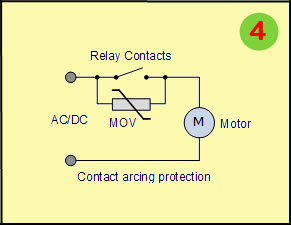Varistor Circuit for Contact Arcing Protection

The varistor circuit shown in the above figure4 represents the contact arcing protection system. In this system, the varistor is connected across the relay contacts that is connected to the motor. The relay is protected from excess voltage transient by a voltage dependent resistor.

Do you know practical application of varistor circuit in real time electronics projects? Then post your views, comments, suggestions, and ideas in the comments section below.

## 2 Comments

1. Mike says:

Thank you for this article!

I was doing a search on VDRs and came upon your article. I have electronic dimmer switches in my house, and am making the move to LED lighting technology. After a year of searching, I finally found some Philips LED downlighters which are dimmable, and thus compatible with my dimmer switches.

The problem I now face is that the very low resistance of the LED replacements falls below the minimum threshold to keep the lights from lighting up in the “off ” position. The specification on the dimmer is 40 Watt minimum for a full “off” (the original R50 globes have a 40 Watt rating). With the four LEDs installed on one circuit, and each LED having a 4W rating, the lights never switch “off” completely.

Could one use a Varistor in the circuit to “fool” the dimmer into switching completely off? Would such a device cause damage to the dimmer switch? I look forward to your reply.

1. Tarun Agarwal says:

Hi Mike,
As we have gone through your comment, but really we are very sorry to inform you that, don’t have the project based on Varistor.
But,we have projects based on LDR (Light Dependent Resistors) and also we suggest you to please follow these below links of two different model numbers,
Model no 221: http://www.efxkits.com/electronic-eye-controlled-security-system
For customization of projects please email us on team@elprocus.com